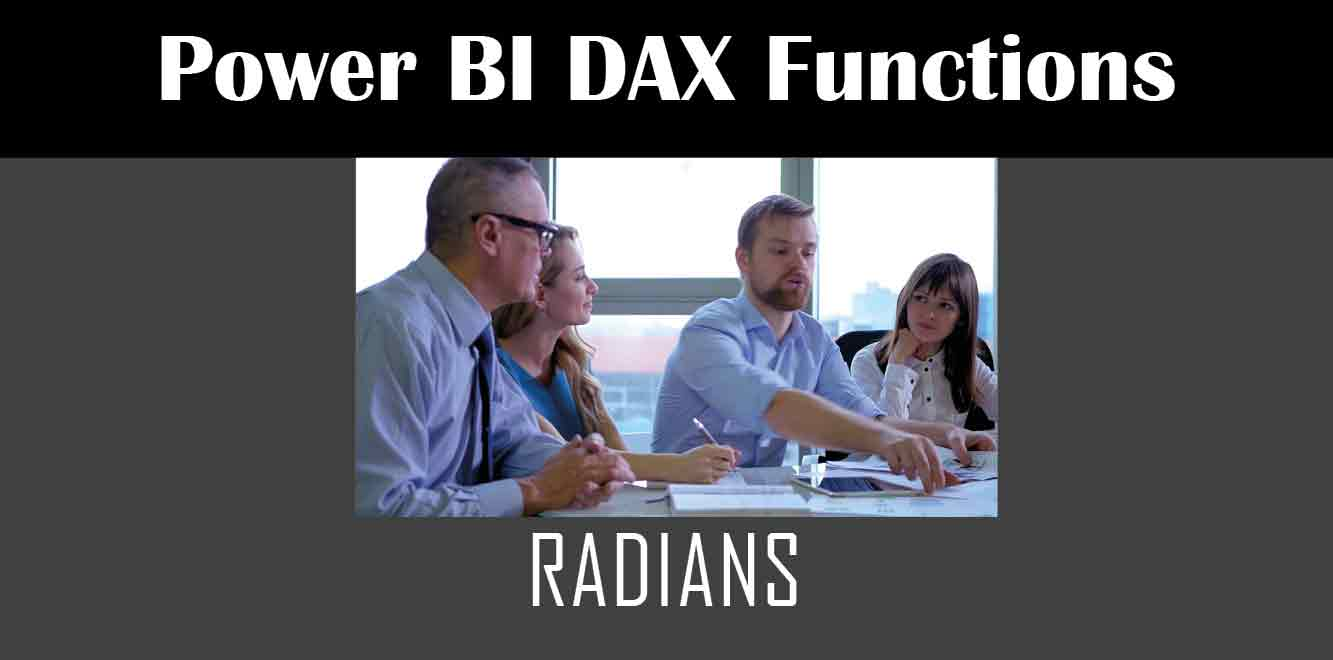## YBefore we dive into the usage of the Power Fx Radians function, it is essential to understand what radians are. Radians are a unit of measurement used in trigonometry to measure angles. Unlike degrees, which use a scale of 360, radians use a scale of 2π, which is approximately 6.28.

The reason radians are so important is that they provide a more natural way to measure angles. They are also used in many mathematical functions, making them a valuable tool in computing applications.

## Syntax of the Power Fx Radians Function

The syntax of the Power Fx Radians function is straightforward. Here is a breakdown of the syntax:

``` The function takes one argument, which is the number you want to convert from degrees to radians. The number is expressed in degrees. Example Usage Here is an example of how to use the Power Fx Radians function in Power Apps. Let's say we want to convert 45 degrees to radians. We would use the following formula: ```

``` This formula would return the value 0.7854, which is the equivalent of 45 degrees in radians. Using the Power Fx Radians Function in Power Apps To use the Power Fx Radians function in Power Apps, you need to follow a few simple steps. Here is a step-by-step guide: 1. Open the Power Apps editor and create a new canvas app. 2. Add a label control to the app screen. 3. Select the label control and navigate to the Properties pane. 4. In the Text field, enter the following formula: ```

``` 5. Run the app, and the label will display the value 0.7854. The Power Fx Radians function is a useful tool in Power Apps. It provides a simple and straightforward way to convert degrees to radians, which is a common calculation used in various applications. By following the steps outlined in this article, you can start using the Power Fx Radians function in your Power Apps projects today. Power Apps Training Courses by G Com Solutions (0800 998 9248)1-Week Intensive Power Apps Training Course £4,975.00 Select optionsContinue Loading DonePower Apps Introduction (2 Days) £1,990.00 Select optionsContinue Loading DonePower Apps Intermediate (1 Day) £995.00 Select optionsContinue Loading DonePower Apps Advanced (2 Days) £1,990.00 Select optionsContinue Loading Done ```
``` ```
``` ```
``` ```
``` ```
``` ```